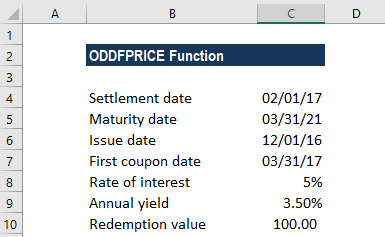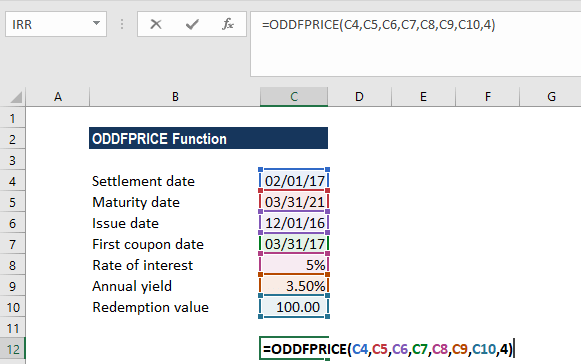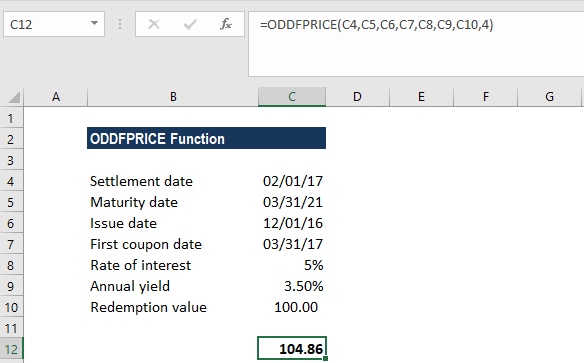# ODDFPRICE Function

Calculates the price per \$100 face value of a security with an odd first period

## What is the ODDFPRICE Function?

The ODDFPRICE Function is an Excel Financial function. It calculates the price per \$100 face value of a security or bond with an odd first period.

In financial analysis, some bonds come with irregular first or last periods. Due to the irregular first or last period, the payment doesn’t fit in any of the usual patterns. If we wish to calculate the price of a bond with an odd first period, we can use the ODDFPRICE function.

### Formula

=ODDFPRICE(Settlement, Maturity, Issue, First_Coupon, Rate, Yld, Redemption, Frequency, [Basis])

The ODDFPRICE function uses the following arguments:

1. Settlement (required argument) – This is the security’s settlement date. It is the date after the issue date when the security is traded to the buyer.
2. Maturity (required argument) – This is the security’s maturity date. It is the date when the security expires.
3. Issue (required argument) – This is the security’s issue date.
4. First_coupon (required argument) – This is the security’s first coupon date.
5. Rate (required argument) – The security’s interest rate.
6. Yld (required argument) – The security’s annual yield.
7. Redemption (required argument) – This is the security’s redemption value per \$100 face value.
8. Frequency (required argument) – This is the number of coupon payments per year. For annual payments, frequency = 1; for semi-annual, frequency = 2; for quarterly, frequency = 4.
9. Basis (optional argument) – This is the type of day count basis to use. The possible values of the basis are as follows:

BasisDay Count basis
0 or omittedUS(NASD) 30/360
1Actual/actual
2Actual/360
3Actual/365
4European 30/360

### How to use the ODDFPRICE Function in Excel?

To understand the uses of the ODDFPRICE function, let’s consider an example:

#### A worked example of using ODDFPRICE.

Let’s assume we need to calculate the price per \$100 face value of a security with issue date Dec. 1, 2016, settlement date Feb. 1, 2017, first coupon date Mar. 31, 2017, and the maturity date Mar. 31, 2021. The rate of interest is 5%, the annual yield is 3.5%, and the redemption value is \$100. Payments are made quarterly and the US (NASD) 30/360 day count basis is used:The formula used is:We get the result below:The above function returned the value 104.86. That is, a security with the above terms would be valued at \$104.86. In the example:

• The date arguments were supplied to the ODDFPRICE function as references to cells containing dates.
• The rate and yield arguments were entered as percentages, 5% and 3.5%, respectively. However, the arguments could instead be entered as the simple numeric values 0.005 and 0.035.
• As we omitted the [basis] argument, the function used the default value 0 (denoting the US (NADS) 30/360 day count basis).

### Notes about the ODDFPRICE Function:

1. #NUM! error – Occurs when:
• The given issue date is greater than or equal to the settlement date.
• Given settlement date is greater than or equal to the first coupon date.
• The first coupon date given is greater than or equal to the maturity date.
• We have provided invalid numbers for the rate, yield, redemption, frequency or [basis] arguments. That is, if either rate is less than 0; yld is less than 0; redemption is less than or equal to 0; frequency is any number other than 1, 2, or 4; or [basis] is any number other than 0, 1, 2, 3, or 4).
2. #VALUE! error – Occurs when:
• The given settlement, maturity, issue or first_coupon arguments are not valid Excel dates.
• Any of the given arguments is non-numeric.

Thanks for reading CFI’s guide to the Excel ODDFPRICE function. By taking the time to learn and master Excel functions, you’ll significantly speed up your financial analysis. To learn more, check out these additional CFI resources:

• Excel Functions for Finance